Courses

# Machine Hour Rate B Com Notes | EduRev

## B Com : Machine Hour Rate B Com Notes | EduRev

The document Machine Hour Rate B Com Notes | EduRev is a part of the B Com Course Cost Accounting.
All you need of B Com at this link: B Com

Machine Hours Rate: Formula, Calculation, Problems and Solutions!
The machine hour rate is similar to the labour hour rate method and is used where the work is performed primarily on machines.

Formula:
The formula used in computing the rate is:

Factory overhead/Machine hours
If factory overhead is Rs 3, 00,000 and total machine hours are 1,500, the machine hour rate is Rs 200 per machine hour (Rs 3, 00,000 ÷ 1500 hours).

Advantages:
This method can be used advantageously where the machine is the major factor in production. In capital intensive industries plants and machines are used in large quantities and one operator may attend to several machines or several operators may attend to single machine. By making the machine the basis, therefore, overhead costs can be equitably absorbed among different products.

Disadvantages:
The disadvantages are:
(1) Machine hour data have to be collected; therefore it requires additional clerical work. The cost of collection and accounting activities goes up and therefore, is not workable for small business firms.
(2) The method cannot be used universally by all business concerns. It can be used where production is mainly through machine. Comparatively, direct labour hours can be used widely by the organizations.

Calculation of Machine Hour Rate:
For the purpose of computing the machine hour rate, each machine (or a group of similar machines) becomes a cost centre and all overheads are charged to a machine cost centre or to differ­ent machine cost centres if many such centres have been created within the department. A machine hour rate for a specific machine cost centre is computed by dividing the total overhead estimated or incurred for that machine divided by actual or estimated machine hours.

The machine hour rate may have different concepts such as:
(1) Ordinary Machine Hour Rate:

This rate takes into account only those overhead expenses which are variable and directly attributed to the running of a machine. Such expenses are power, fuel, repair, maintenance and depreciation. The total of all these expenses is divided by the total machine hours.

(2) Composite Machine Hour Rate:
This method takes into account not only expenses directly connected with the machine as mentioned above, but also other expenses which are known as stand­ing or fixed charges. Such expenses are rent and rates, supervisory, labour, lighting and heating, etc. These expenses being fixed in nature are determined for a particular period and then apportioned among different departments on some equitable bases. The overhead expenses thus apportioned to each department are further apportioned among the machines (machine cost centres) in that depart­ment on an equitable basis.
The following are bases used for the apportionment of expenses for computing machine hour rate: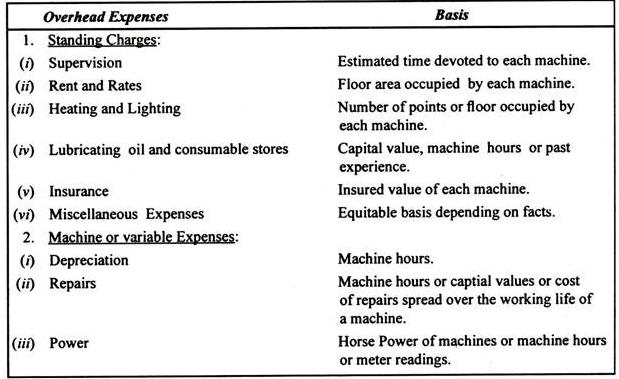Selecting an Absorption Rate:
The above absorption rates have their own merits and demerits. The method to be used depends on the factors and circumstances prevailing in a manufacturing firm.
Whatever method is selected by a firm, it must achieve the following objectives:
(1) The basic objective is to select an absorption base which helps in determining the accurate amount of factory overhead to be charged to individual products, jobs, processes, etc. The base should have cause and effect relationship with the incurrence of the overhead costs and should be physically related to the production of the products. If overhead items mainly relate to the use of machinery, a machine hour rate will be suitable. If overhead items originate due to purchasing and handling materials, then the materials cost may be selected as the base.
(2) A secondary objective in selecting a method of absorption is to minimise clerical effort and cost. When two or more absorption rates tend to charge the same amount of overhead, the simplest base could be used. Generally speaking, the direct labour cost or materials cost base do involve least clerical effort and cost, whereas direct labour hour and machine hour involve more clerical work and are expensive. In a standard costing system the usual bases are standard direct labour cost, standard labour hours, and standard machine hours.
(3) The selection of an absorption base is also influenced by other factors, such as type of industry, legal requirements, if any, policy of management, etc. in addition to the suitability of a method under specific circumstances.

Illustrative Problem 1:
The Genetry Company produces two products, X and Y.
Estimated costs are presented below for a year in which 12,500 units of each product are expected to be sold: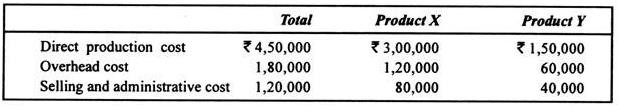An annual profit of Rs 1, 50,000 for the whole company is considered satisfactory. The com­pany uses the same gross margin percentage (i.e., mark-on) to arrive at the price for both products.
Required:
(a) Calculate selling prices for both products X and Y.
(b) Using the prices calculated above, how much profit would result if sales were 15,000 units of X and 10,000 units of Y instead of 12,500 units of each?
(c) Comment on the effect of changes in the product mix on total profit when the same margin percentage is used (M Com., Delhi 1993)
Solution:
Determination of selling price: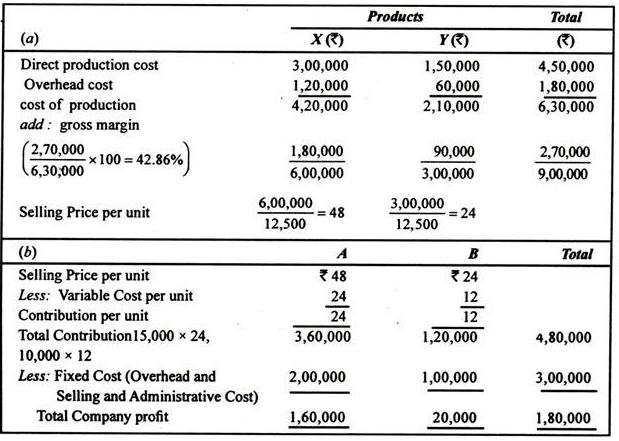(c) The total company profit is Rs 180,000 in situation (B) as compared to Rs 1, 50,000 in (A)
Note:
Gross profit will be Rs 2, 70,000 as total profit is Rs 1, 50,000 after paying selling and administrative cost of Rs 1, 20,000. Thus,
Gross profit = Selling and Admn. + Company profit
= 1, 20,000 + 1, 50,000 = Rs 2, 70,000.
Variable cost per unit = X, (3, 00, 000/12, 500) = Rs 24, and Y, (1, 50, 000/12, 500) = Rs 12

Illustrative Problem 2:
Topside Limited has two production departments A and B and two service departments— Fac­tory Office and Materials Handling. All factory overhead costs are traceable to departments with the exception of building occupancy.
According to the managerial policy, Department A has to absorb factory overhead on a machine- hour basis and Department B has to absorb them on direct labour hour basis.
At normal capacity of 10,000 machine hours in Department A and of 20,000 direct labour hours in department B, the budgeted allowances for the direct department costs for a year are as follows: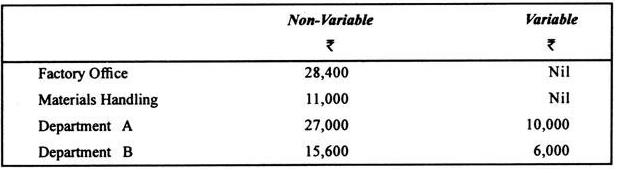Building occupancy costs have been budgeted at Rs. 20,000 per year and are non-variable with volume.
The following data concerning production operation have been made available: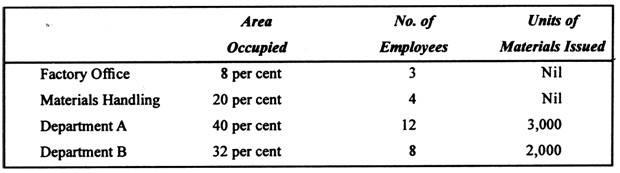You are required to:
(i) Prepare on Overhead Distribution Summary departmentalizing factory overhead costs.
(ii) Determine departmental non -variable and variable absorption rates at normal capacity (M.Com, Delhi 1995)
Solution.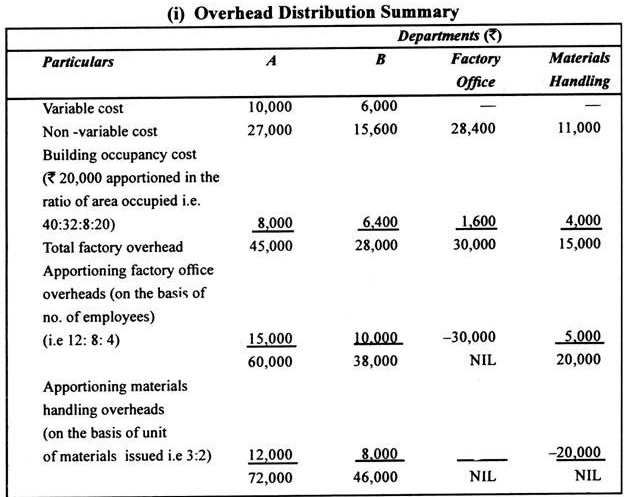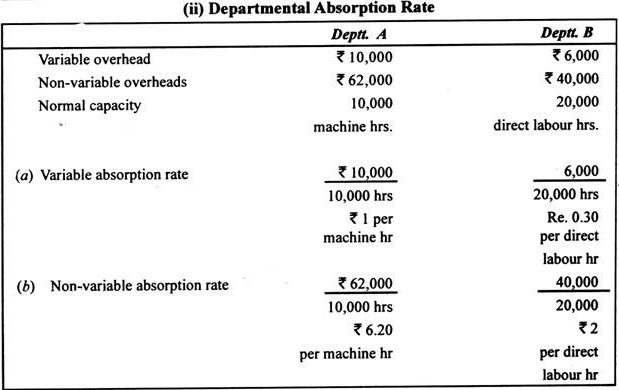Illustrative Problem 3:
In a machine shop, the machine hour rate is worked out at the beginning of a year on the basis of a 13-week period which is equal to three calendar months.
The Following estimates for operating a machine are relevant: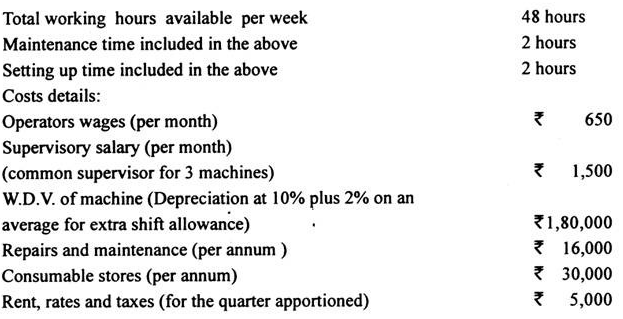Power consumed is @ 15 units per hour @ 40 paise per unit. Power is required for productive hours only, setting -up time is part of productive time but no power is required for setting up jobs.
The operator and supervisor are permanent. Repairs and maintenance and consumable stores are variable.
You are required to:
(a) Work out the machine hour rate.
(b) Work out the rate for quoting to the outside party for utilising the ideal capacity in the machine shop assuming a profit of 20% above variable cost.
Solution.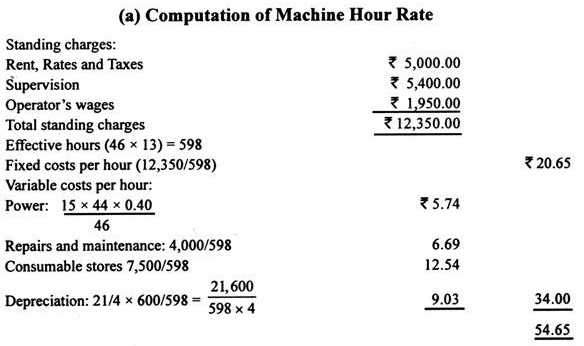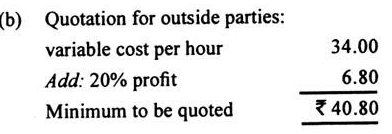Illustrative Problem 4:
An engineering company, engaged in the manufacturing of various heavy engineering products, has installed one Pegard Numerical Control Horizontal Borer for specialised manufacturing opera­tions.
Calculate the machine hour rate on the basis of the following particulars:
(i) F.O.B. cost of the machine Rs 24 lakhs
(ii) Customs duty, insurance, freight, etc. Rs 11 lakhs.
(iii) Installation expenses Rs 3 lakh
(iv) Cost of tools adequate for 2 years only Rs 4 lakhs,
(v) Cost of machine room Rs 3 lakhs
(vi) Cost of air conditioning for machine room Rs 2 lakhs,
(vii) Rate of interest on term loan to finance the above capital expenditure 12% per annum
(viii) Salaries, etc. for operators and supervisory staff Rs 2 lakhs per year
(ix) Cost of electricity Rs 11 per hour
(x) Consumption of stores Rs 5000 per month
(xi) Other expenses Rs 5 lakh per annum
(xii) Assume rate of depreciation as 10% per annum on fixed assets,
(xiii) Total working hours in the machine room is 200 hours in a month
(xiv) Loading and unloading time is 10% of machine time,
(xv) You can make suitable as­sumptions if necessary, for the purpose of your computation.
Solution.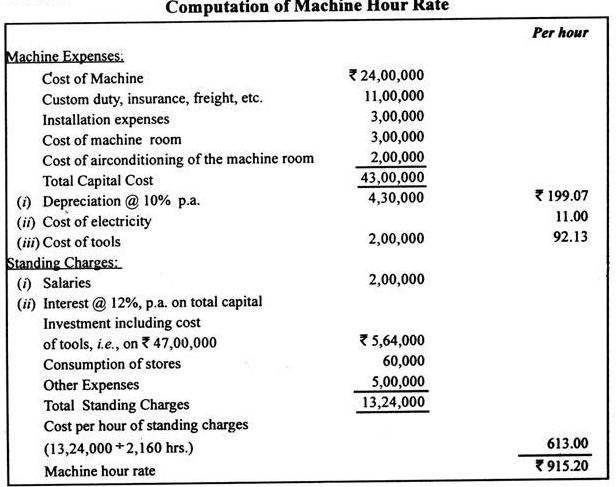Notes:
(1) Total working hours per annum are 200 x 12 = 2,400.
(2) Loading and unloading time is 10% of machine time.
(3) Effective working hours are 2,160 hours (2,400 – 10% of 2,400).
(4) It is assumed that there is no consumption of electricity during loading and unloading time.
(5) Interest has been treated as an element of cost. It could be ignored also.

Offer running on EduRev: Apply code STAYHOME200 to get INR 200 off on our premium plan EduRev Infinity!

## Cost Accounting

131 videos|139 docs

,

,

,

,

,

,

,

,

,

,

,

,

,

,

,

,

,

,

,

,

,

;